Courses

# Coordinate Geometry - Important Formulae of Number system - Free CAT Notes | EduRev

## CAT : Coordinate Geometry - Important Formulae of Number system - Free CAT Notes | EduRev

The document Coordinate Geometry - Important Formulae of Number system - Free CAT Notes | EduRev is a part of the CAT Course Quantitative Aptitude (Quant).
All you need of CAT at this link: CAT
• Distance between two points P(x1,y1) and Q(x2,y2) is given by =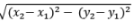• If a point R (x,y) divides P(x1,y1) and Q(x2,y2) internally in the ratio of m:n, the coordinates of R ie (x,y) are given by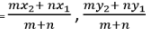• If a point R (x,y) divides P(x1,y1) and Q(x2,y2) externally in the ratio of m:n, the coordinates of R ie (x,y) are given by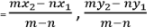EduRev's Tip:

•  The X axis divides the line joining P(x1,y1) and Q(x2,y2) in the ratio of y1 : y2
• The Y axis divides the line joining P(x1,y1) and Q(x2,y2) in the ratio of x1 : x2

Slope(m) of a line is the tangent of the angle made by the line with the positive direction of the X-Axis.
For a general equation ax + by + c = 0; slope (m) = -a/b.
For a line joining two points, P (x1,y1) and Q(x2,y2), the slope(m) is =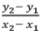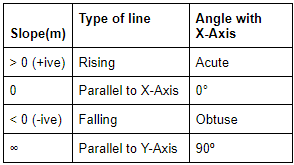Equation of a line parallel to X-axis is y = a {Of X-Axis is y = 0}
Equation of a line parallel to Y-Axis is x = a {Of Y-Axis is x = 0}
The intercept of a line is the distance between the point where it cuts the X-Axis or Y-Axis and the origin. Y-Intercept is often denoted with the letter ‘c’.

Equation of a line
General form: ax + by + c = 0

Slope Intercept Form: Slope is m, y-intercept is c
⇒ y = mx + c

Slope Point Form: Slope is m, point is x1,y1
⇒ y – y1 = m(x – x1)

Two Point Form: Two points are x1,y1 and x2,y2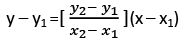Two Intercept Form: X-intercept is a, Y-intercept is b.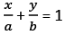OR bx + ay = ab
A cute angle between two lines with slope m1 and m2 is given by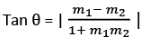⇒ For parallel lines, θ = 0°;  m1 = m2
⇒ For parallel lines, θ = 90°; m1m2 = -1
Distance of a point P (x1,y1) from a line ax + by + c = 0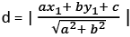⇒ From origin, d =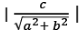Distance between two parallel lines, ax + by + c1 = 0 and ax + by + c2 = 0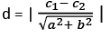EduRev's Tip: If we know three points A(x1,y1), B(x2,y2 ) and C(x2,y2)  of a parallelogram, the fourth point is given by
⇒ (x1 + x3 – x2, y1 + y3 – y2)

Triangle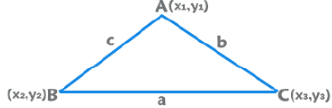The vertices are P (x1,y1), Q(x2,y2) and R(x3,y3)
Incenter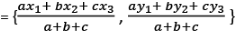Centroid =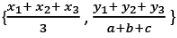Area = ½ [ x1(y2 – y3) + x2 (y3 – y1) + x3 (y1 – y2)]

Circle
General Equation: x2 + y2 + 2gx + 2fy + c = 0
⇒ Centre is (-g, -f) and radius =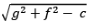Centre is (h, k) and radius is r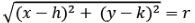Centre is origin and radius is r
⇒ x2 + y2 = r2

Offer running on EduRev: Apply code STAYHOME200 to get INR 200 off on our premium plan EduRev Infinity!

## Quantitative Aptitude (Quant)

116 videos|131 docs|131 tests

,

,

,

,

,

,

,

,

,

,

,

,

,

,

,

,

,

,

,

,

,

;